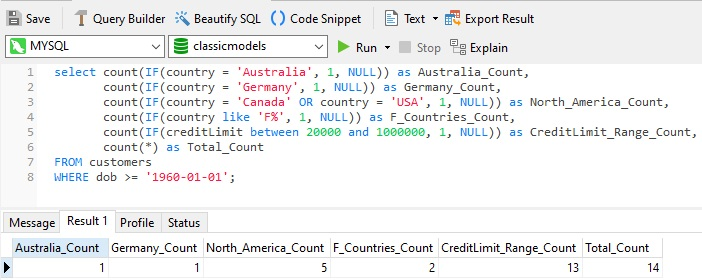# Navicat Blog

Null Values and the SQL Count() Function Oct 25, 2021 by Robert Gravelle

Back in March of 2020, the The NULL Value and its Purpose in Relational Database Systems article presented the NULL value and its special meaning in relational databases. That article also described how to allow NULLs in your database tables and how to reference them in queries. In today's blog, we'll learn how to combine NULLs with the SQL Count() function to achieve a variety of objectives.

# Counting Null and Non-null Values

The Count() function comes in two flavors: COUNT(*) returns all rows in the table, whereas COUNT(Expression) ignores Null expressions. Hence, if you provide a column name that allows NULL values, then Count() will return all rows that have a non-null value. These two separate uses of Count() provide an important clue as to how we can obtain a count of NULL values for a specific column. And that is by subtracting the non-NULL fields from the Total fields, like so:

```SELECT COUNT(*) - COUNT(<Column Name>)
```

Now that we know how to count null, non-null, and all rows in a table, let's see an example. We'll run this query against the customers table of the MySQL classicmodels Sample Database. Here is that table in Navicat Premium's Table Designer:The addressline2 field contains additional address details that are not part of the street name and number. Hence, it's not required for all addresses, as we can see in this sample of table data:This query uses the Count() function in three ways to show all table rows, the number of populated addressLine2 rows and Nulls:

```SELECT COUNT(*) AS All_Rows,
FROM customers;
```

Here is the above SELECT statement in Navicat Premium's Query Designer, along with the results:# Using NULL in Content Analytics

That fact that the COUNT(Expression) version of the Count() function ignores Null expressions can be extremely helpful in compiling statistics about table data, especially when combined with other functions such as the SQL IF() function, which is basically the SQL equivalent of the Ternary Operator:

```IF(predicate, true-value, false-value)
```

If the predicate is true, IF evaluates to the true-value, or 1 in the query below. If the predicate is false, it evaluates to the false-value, or NULL, as seen in the statement below. The COUNTs then tabulate each row where the IFs evaluate to 1, i.e., where the predicate is true:

```SELECT count(IF(country = 'Australia', 1, NULL)) as Australia_Count,
count(IF(country = 'Germany', 1, NULL)) as Germany_Count,
count(IF(country = 'Canada' OR country = 'USA', 1, NULL)) as North_America_Count,
count(IF(country like 'F%', 1, NULL)) as F_Countries_Count,
count(IF(creditLimit between 20000 and 1000000, 1, NULL)) as CreditLimit_Range_Count,
count(*) as Total_Count
FROM customers
WHERE dob >= '1960-01-01';
```

Here is the query and results in Navicat:# Conclusion

In today's blog, we learned how to combine NULLs with the SQL Count() function to achieve a variety of objectives. More than a way to count NULL and non-NULL values, when combined with other SQL function such as IF() and SUM(), these can be utilized to compile all sorts of statistics on your data!

Blog Archives
Share
• Key Topics• Products• Partners